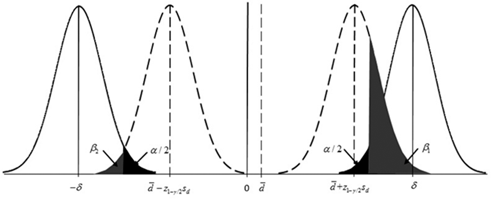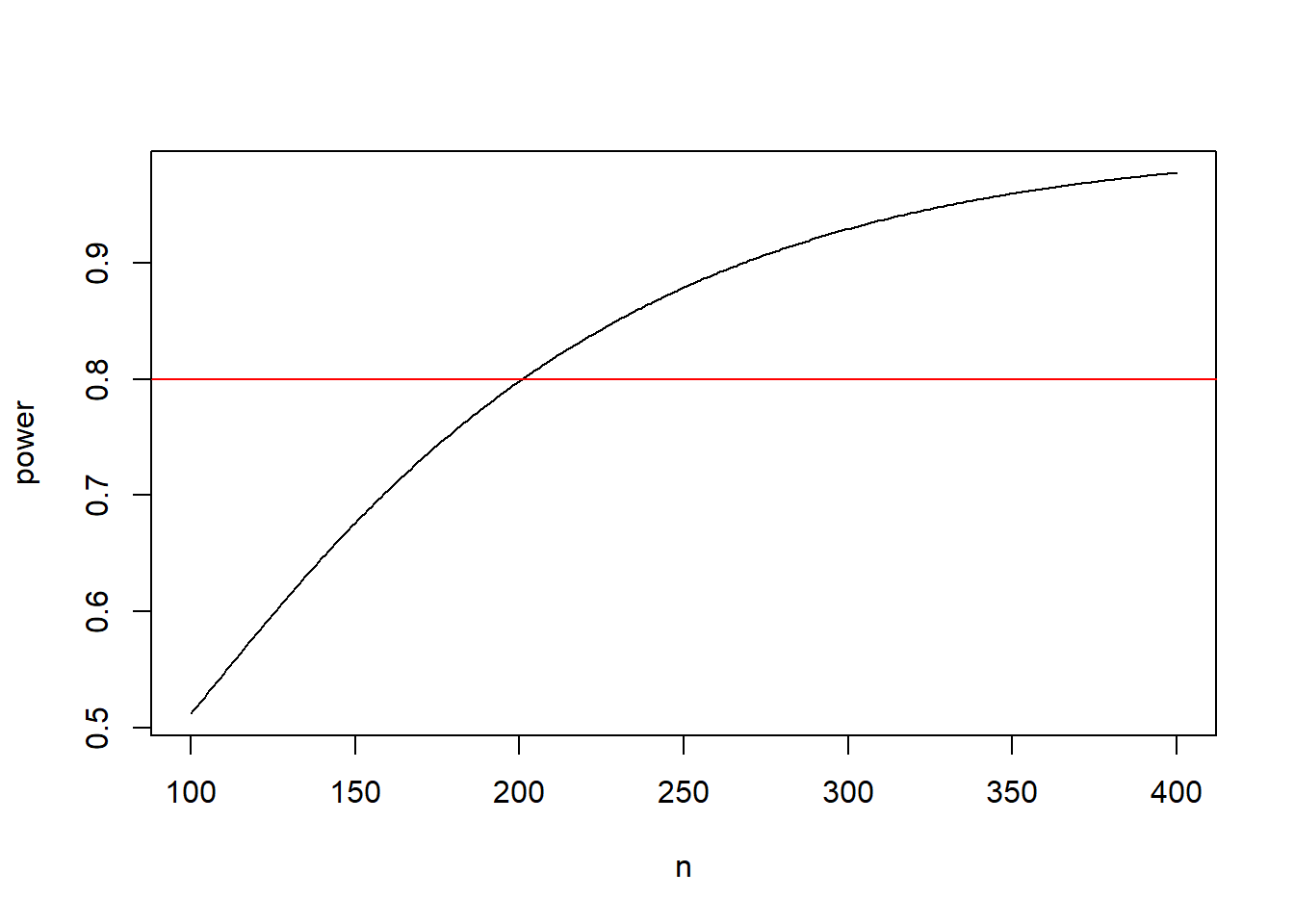## Motivation

I was recently approached by a PI to complete sample size and power calculations for Bland-Altman agreement assessment using the Lu et al (2016) formulation. The goal of this short tutorial is to demonstrate how to perform quick sample size calculations in R following Lu et al (2016).

## Limits of agreement (LOAs)

Let $$D$$ be the random variable representing the difference between two sets of measurements $$X$$ and $$Y$$. For a sample of $$d_i = x_i - y_i$$, $$i=1,\dots,n$$ with mean $$\bar{d}$$ and standard deviation $$s_d$$, the $$100(1- \gamma)\%$$ LOAs can be calculated as $$\bar{d} \pm z_{1-\gamma/2}s_d$$, which yields an upper limit and a lower limit of LOA.

The $$100(1- \alpha)\%$$ confidence interval estimation of the $$100(1- \gamma)\%$$ LOAs can be obtained following

$Lower bound = (\bar{d} - z_{1-\gamma/2}s_d) - t_{1-\alpha/2, df=n-1} s_d \sqrt{\frac{1}{n}+\frac{z^2_{1-\gamma/2}s_d}{2(n-1)}}$ $Upper bound = (\bar{d} + z_{1-\gamma/2}s_d) + t_{1-\alpha/2, df=n-1} s_d \sqrt{\frac{1}{n}+\frac{z^2_{1-\gamma/2}s_d}{2(n-1)}}.$

We can proceed with sample size calculation by pre-specifying i) a desired confidence band/limit of the LOAs and ii) the two significant levels $$\alpha$$ and $$\gamma$$ (e.g. commonly set at 0.05).

The confidence interval of LOAs can be assumed symmetric. The decomposition of the type I and II errors of LOAs is shown in the figure below (taken directly from the paper). The two type II error terms, $$\beta_1$$ and $$\beta_2$$ can be calculated following a non-central t-distribution,Figure 1, from Lu et al (2016).

where $$\beta_1 = 1 - probt[t_{1-\alpha/2, n-1}, n-1, \tau_1 ]$$ and $$\beta_2 = 1 - probt[t_{1-\alpha/2, n-1}, n-1, \tau_2 ]$$ and $$\tau_1$$ and $$\tau_2$$ are the two non-central distribution parameters. For mathematical details including the definition of $$\tau_1$$ and $$\tau_2$$ and the final power formula please see Lu et al (2016).

## Power Calculation

For power calculation under a given sample size, we specify the following parameters

• $$\bar{d}$$, mean difference between the two sets of measures
• $$s_d$$, standard deviation of the difference
• $$\delta$$, targeted clinical agreement limit
• $$n$$, available sample size
• $$\alpha$$, targeted confidence level of CIs of LOAs
• $$\beta$$, targeted confidence level of LOA

Example 1. We have $$\bar{d} = 0.2$$, $$s_d=1$$, $$\delta=2.5$$, $$n=203$$ and $$\alpha = \beta = 0.05$$. The following R code returns the power estimate using Lu et al (2016). Under this setting, the study has 80% power.

# attempt to verify Lu et al (2016) example;
# https://doi.org/10.1515/ijb-2015-0039;
mud = 0.2 #mean of difference;
sd = 1 #expected sd of the difference;
delta= 2.5 #targeted clinical agreement limit;
n = 203 #available sample size;

alpha = 0.05 # 95% confidence level of CIs of LoAs;
gamma = 0.05 # 95% confidence level of LoA;

# non-centrality parameter tau1 and tau2;
tau1 = (delta - mud - qnorm(1-gamma/2)*sd)/(sd*sqrt(1/n+qnorm(1-gamma/2)^2/(2*(n-1))))
tau2 = (delta + mud - qnorm(1-gamma/2)*sd)/(sd*sqrt(1/n+qnorm(1-gamma/2)^2/(2*(n-1))))

power = pt(qt(1-alpha/2,n-1), n-1, ncp=tau1, lower.tail = FALSE)+pt(qt(1-alpha/2,n-1), n-1, ncp=tau2, lower.tail = TRUE)

#return power estimate;
power
##  0.8042332

## Sample Size Calculation

Example 2. We can extend the code above to return power calculation giving a sequence of sample sizes. Let’s say we are interested to identify sample sizes corresponding to 70% and 90% power.

The R code below produces a simple power curve and returns the required sample size to reach 70%, 80% and 90% power.

# attempt to verify Lu et al (2016) example;
# https://doi.org/10.1515/ijb-2015-0039;
mud = 0.2 #mean of difference;
sd = 1 #expected sd of the difference;
delta= 2.5 #targeted clinical agreement limit;
n = seq(100,400,1) #sample size range between 100 to 400;

alpha = 0.05 # 95% confidence level of CIs of LoAs;
gamma = 0.05 # 95% confidence level of LoA;

# non-centrality parameter tau1 and tau2;
tau1 = (delta - mud - qnorm(1-gamma/2)*sd)/(sd*sqrt(1/n+qnorm(1-gamma/2)^2/(2*(n-1))))
tau2 = (delta + mud - qnorm(1-gamma/2)*sd)/(sd*sqrt(1/n+qnorm(1-gamma/2)^2/(2*(n-1))))

power = pt(qt(1-alpha/2,n-1), n-1, ncp=tau1, lower.tail = FALSE)+pt(qt(1-alpha/2,n-1), n-1, ncp=tau2, lower.tail = TRUE)

results<-cbind(n, power)

# view sample size and power table;
##        n     power
## [1,] 100 0.5118853
## [2,] 101 0.5153101
## [3,] 102 0.5187459
## [4,] 103 0.5221911
## [5,] 104 0.5256439
## [6,] 105 0.5291028

# plot power curve;
plot(n, power, type = "l")
abline(h = 0.8, col = "red")# return sample size at 70%, 80% and 90% power;
# for this calculation we select the sample size that yields the smallest value over the target power threshold.
rbind(results[min(which(results[,2] > 0.7)),],
results[min(which(results[,2] > 0.8)),],
results[min(which(results[,2] > 0.9)),])
##        n     power
## [1,] 159 0.7016833
## [2,] 201 0.8003178
## [3,] 269 0.9010620

The minimum required sample size to reach 70% power is 159 and minimum required sample size to reach 90% power is 269.